Next: Third Adiabatic Invariant Up: Charged Particle Motion Previous: Ring Current

We have seen that there is an adiabatic invariant associated with the periodic gyration of a charged particle around magnetic field-lines. Thus, it is reasonable to suppose that there is a second adiabatic invariant associated with the periodic bouncing motion of a particle trapped between two mirror points on a magnetic field-line. This is indeed the case.

Recall that an adiabatic invariant is the lowest order approximation to a Poincaré invariant: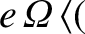(145)

In this case, let the curve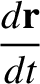correspond to the trajectory of a guiding centre as a charged particle trapped in the Earth's magnetic field executes a bounce orbit. Of course, this trajectory does not quite close, because of the slow azimuthal drift of particles around the Earth. However, it is easily demonstrated that the azimuthal displacement of the end point of the trajectory, with respect to the beginning point, is of order the gyroradius. Thus, in the limit in which the ratio of the gyroradius,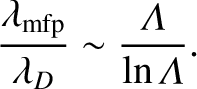, to the variation length-scale of the magnetic field,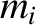, tends to zero, the trajectory of the guiding centre can be regarded as being approximately closed, and the actual particle trajectory conforms very closely to that of the guiding centre. Thus, the adiabatic invariant associated with the bounce motion can be written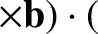(146)

where the path of integration is along a field-line: from the equator to the upper mirror point, back along the field-line to the lower mirror point, and then back to the equator. Furthermore,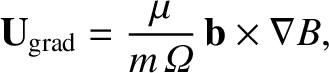is an element of arc-length along the field-line, and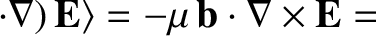. Using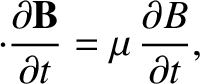, the above expression yields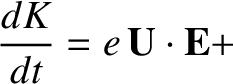(147)

Here,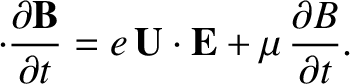is the total magnetic flux enclosed by the curve--which, in this case, is obviously zero. Thus, the so-called second adiabatic invariant or longitudinal adiabatic invariant takes the form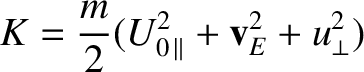(148)

In other words, the second invariant is proportional to the loop integral of the parallel (to the magnetic field) velocity taken over a bounce orbit. Actually, the above proof'' is not particularly rigorous: the rigorous proof that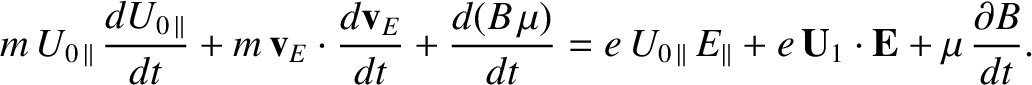is an adiabatic invariant was first given by Northrop and Teller.It should be noted, of course, thatis only a constant of the motion for particles trapped in the inner magnetosphere provided that the magnetospheric magnetic field varies on time-scales much longer than the bounce time,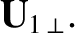. Since the bounce time for MeV energy protons and electrons is, at most, a few seconds, this is not a particularly onerous constraint.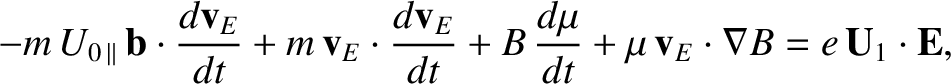The invariance ofis of great importance for charged particle dynamics in the Earth's inner magnetosphere. It turns out that the Earth's magnetic field is distorted from pure axisymmetry by the action of the solar wind, as illustrated in Fig. 4. Because of this asymmetry, there is no particular reason to believe that a particle will return to its earlier trajectory as it makes a full rotation around the Earth. In other words, the particle may well end up on a different field-line when it returns to the same azimuthal angle. However, at a given azimuthal angle, each field-line has a different length between mirror points, and a different variation of the field-strength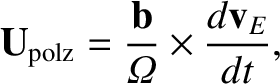between the mirror points, for a particle with given energy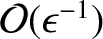and magnetic moment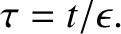. Thus, each field-line represents a different value offor that particle. So, ifis conserved, as well asand, then the particle must return to the same field-line after precessing around the Earth. In other words, the conservation ofprevents charged particles from spiraling radially in or out of the Van Allen belts as they rotate around the Earth. This helps to explain the persistence of these belts.Next: Third Adiabatic Invariant Up: Charged Particle Motion Previous: Ring Current
Richard Fitzpatrick 2011-03-31